# NCERT solutions for Class 7 Maths chapter 14 - Symmetry [Latest edition]

#### Chapters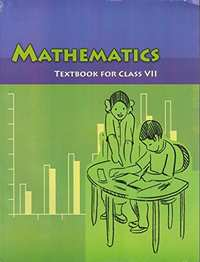## Chapter 14: Symmetry

Exercise 14.1Exercise 14.2Exercise 14.3
Exercise 14.1 [Pages 268 - 270]

### NCERT solutions for Class 7 Maths Chapter 14 Symmetry Exercise 14.1 [Pages 268 - 270]

Exercise 14.1 | Q 1.01 | Page 268

Copy the figure with punched holes and find the axes of symmetry for the following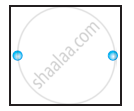Exercise 14.1 | Q 1.02 | Page 268

Copy the figure with punched holes and find the axes of symmetry for the following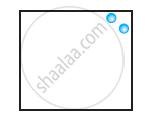Exercise 14.1 | Q 1.03 | Page 268

Copy the figure with punched holes and find the axes of symmetry for the following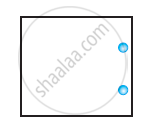Exercise 14.1 | Q 1.04 | Page 268

Copy the figure with punched holes and find the axes of symmetry for the following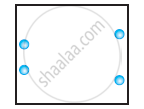Exercise 14.1 | Q 1.05 | Page 268

Copy the figure with punched holes and find the axes of symmetry for the following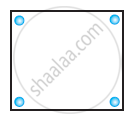Exercise 14.1 | Q 1.06 | Page 268

Copy the figure with punched holes and find the axes of symmetry for the following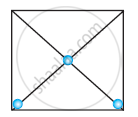Exercise 14.1 | Q 1.07 | Page 268

Copy the figure with punched holes and find the axes of symmetry for the following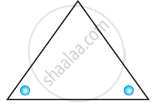Exercise 14.1 | Q 1.08 | Page 268

Copy the figure with punched holes and find the axes of symmetry for the following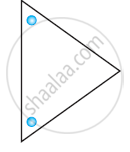Exercise 14.1 | Q 1.09 | Page 269

Copy the figure with punched holes and find the axes of symmetry for the following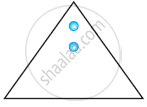Exercise 14.1 | Q 1.1 | Page 268

Copy the figure with punched holes and find the axes of symmetry for the following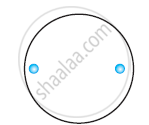Exercise 14.1 | Q 1.11 | Page 268

Copy the figure with punched holes and find the axes of symmetry for the following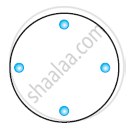Exercise 14.1 | Q 1.12 | Page 268

Copy the figure with punched holes and find the axes of symmetry for the following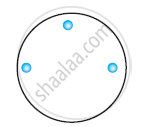Exercise 14.1 | Q 2.05 | Page 269

Given the line(s) of symmetry, find the other hole(s):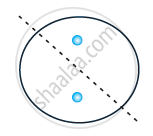Exercise 14.1 | Q 2.1 | Page 269

Given the line(s) of symmetry, find the other hole(s):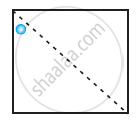Exercise 14.1 | Q 2.2 | Page 269

Given the line(s) of symmetry, find the other hole(s):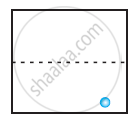Exercise 14.1 | Q 2.3 | Page 269

Given the line(s) of symmetry, find the other hole(s):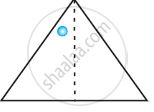Exercise 14.1 | Q 2.4 | Page 268

Given the line(s) of symmetry, find the other hole(s):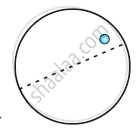Exercise 14.1 | Q 3.1 | Page 269

In the given figure, the mirror line (i.e., the line of symmetry) is given as a dotted line. Complete given figure performing reflection in the dotted (mirror) line. (You might perhaps place a mirror along the dotted line and look into the mirror for the image). Are you able to recall the name of the figure you complete?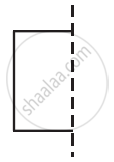Exercise 14.1 | Q 3.2 | Page 269

In the given figure, the mirror line (i.e., the line of symmetry) is given as a dotted line. Complete given figure performing reflection in the dotted (mirror) line. (You might perhaps place a mirror along the dotted line and look into the mirror for the image). Are you able to recall the name of the figure you complete?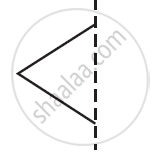Exercise 14.1 | Q 3.3 | Page 269

In the given figure, the mirror line (i.e., the line of symmetry) is given as a dotted line. Complete given figure performing reflection in the dotted (mirror) line. (You might perhaps place a mirror along the dotted line and look into the mirror for the image). Are you able to recall the name of the figure you complete?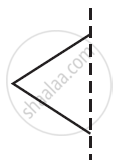Exercise 14.1 | Q 3.4 | Page 269

In the given figure, the mirror line (i.e., the line of symmetry) is given as a dotted line. Complete given figure performing reflection in the dotted (mirror) line. (You might perhaps place a mirror along the dotted line and look into the mirror for the image). Are you able to recall the name of the figure you complete?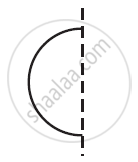Exercise 14.1 | Q 3.5 | Page 269

In the given figure, the mirror line (i.e., the line of symmetry) is given as a dotted line. Complete given figure performing reflection in the dotted (mirror) line. (You might perhaps place a mirror along the dotted line and look into the mirror for the image). Are you able to recall the name of the figure you complete?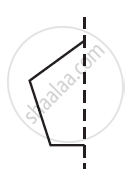Exercise 14.1 | Q 3.6 | Page 269

In the given figure, the mirror line (i.e., the line of symmetry) is given as a dotted line. Complete given figure performing reflection in the dotted (mirror) line. (You might perhaps place a mirror along the dotted line and look into the mirror for the image). Are you able to recall the name of the figure you complete?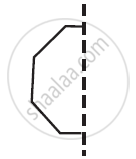Exercise 14.1 | Q 4.1 | Page 269

The following figures have more than one line of symmetry. Such figures are said to have multiple lines of symmetry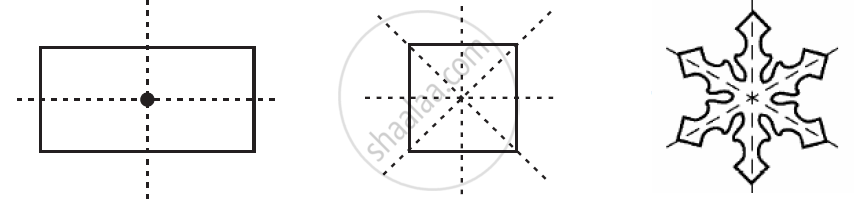Identify multiple lines of symmetry, if any, in the given figure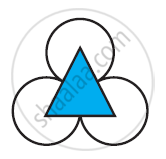Exercise 14.1 | Q 4.2 | Page 269

The following figures have more than one line of symmetry. Such figures are said to have multiple lines of symmetryIdentify multiple lines of symmetry, if any, in the given figure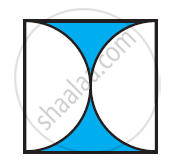Exercise 14.1 | Q 4.3 | Page 269

The following figures have more than one line of symmetry. Such figures are said to have multiple lines of symmetryIdentify multiple lines of symmetry, if any, in the given figure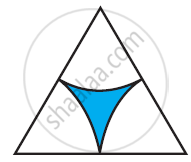Exercise 14.1 | Q 4.4 | Page 269

The following figures have more than one line of symmetry. Such figures are said to have multiple lines of symmetryIdentify multiple lines of symmetry, if any, in the given figure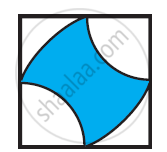Exercise 14.1 | Q 4.5 | Page 270

The following figures have more than one line of symmetry. Such figures are said to have multiple lines of symmetryIdentify multiple lines of symmetry, if any, in the given figure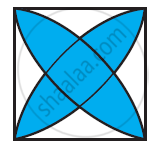Exercise 14.1 | Q 4.6 | Page 270

The following figures have more than one line of symmetry. Such figures are said to have multiple lines of symmetryIdentify multiple lines of symmetry, if any, in the given figure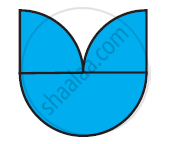Exercise 14.1 | Q 4.7 | Page 270

The following figures have more than one line of symmetry. Such figures are said to have multiple lines of symmetryIdentify multiple lines of symmetry, if any, in the given figure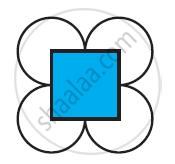Exercise 14.1 | Q 4.8 | Page 270

The following figures have more than one line of symmetry. Such figures are said to have multiple lines of symmetryIdentify multiple lines of symmetry, if any, in the given figure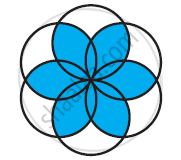Exercise 14.1 | Q 5 | Page 270

Copy the figure given hereTake any one diagonal as a line of symmetry and shade a few more squares to make the figure symmetric about a diagonal. Is there more than one way to do that? Will the figure be symmetric about both the diagonals?

Exercise 14.1 | Q 6.1 | Page 270

Copy the diagram and complete the given shape to be symmetric about the mirror line(s):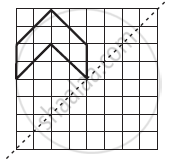Exercise 14.1 | Q 6.2 | Page 270

Copy the diagram and complete the given shape to be symmetric about the mirror line(s):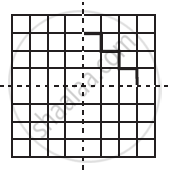Exercise 14.1 | Q 6.3 | Page 270

Copy the diagram and complete the given shape to be symmetric about the mirror line(s):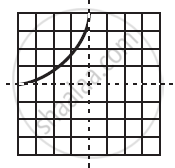Exercise 14.1 | Q 7.01 | Page 270

State the number of lines of symmetry for An equilateral triangle figure

Exercise 14.1 | Q 7.02 | Page 270

State the number of lines of symmetry for An isosceles triangle figure

Exercise 14.1 | Q 7.03 | Page 270

State the number of lines of symmetry for A scalene triangle figure

Exercise 14.1 | Q 7.04 | Page 270

State the number of lines of symmetry for A square figure

Exercise 14.1 | Q 7.05 | Page 270

State the number of lines of symmetry for A rectangle figure

Exercise 14.1 | Q 7.06 | Page 270

State the number of lines of symmetry for A rhombus figure

Exercise 14.1 | Q 7.07 | Page 270

State the number of lines of symmetry for A parallelogram figure

Exercise 14.1 | Q 7.08 | Page 270

State the number of lines of symmetry for A quadrilateral figure

Exercise 14.1 | Q 7.09 | Page 270

State the number of lines of symmetry for A regular hexagon figure

Exercise 14.1 | Q 7.1 | Page 270

State the number of lines of symmetry for A circle figure

Exercise 14.1 | Q 8.1 | Page 270

What letters of the English alphabet have reflectional symmetry (i.e., symmetry related to mirror reflection) about a vertical mirror

Exercise 14.1 | Q 8.2 | Page 270

What letters of the English alphabet have reflectional symmetry (i.e., symmetry related to mirror reflection) about a horizontal mirror

Exercise 14.1 | Q 8.3 | Page 270

What letters of the English alphabet have reflectional symmetry (i.e., symmetry related to mirror reflection) about both horizontal and vertical mirrors

Exercise 14.1 | Q 9 | Page 270

Give three examples of shapes with no line of symmetry.

Exercise 14.1 | Q 10.1 | Page 270

What other name can you give to the line of symmetry of an isosceles triangle?

Exercise 14.1 | Q 10.2 | Page 270

What other name can you give to the line of symmetry of a circle?

Exercise 14.2 [Page 274]

### NCERT solutions for Class 7 Maths Chapter 14 Symmetry Exercise 14.2 [Page 274]

Exercise 14.2 | Q 1.1 | Page 274

The following figure have rotational symmetry of order more than 1: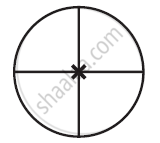Exercise 14.2 | Q 1.2 | Page 274

The following figure have rotational symmetry of order more than 1: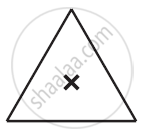Exercise 14.2 | Q 1.3 | Page 274

The following figure have rotational symmetry of order more than 1: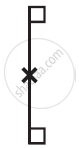Exercise 14.2 | Q 1.4 | Page 274

The following figure have rotational symmetry of order more than 1: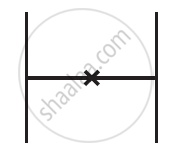Exercise 14.2 | Q 1.5 | Page 274

The following figure have rotational symmetry of order more than 1: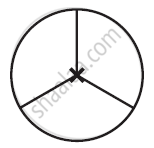Exercise 14.2 | Q 1.6 | Page 274

The following figure have rotational symmetry of order more than 1: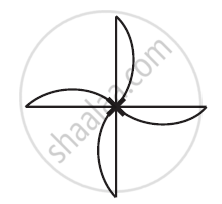Exercise 14.2 | Q 2.1 | Page 274

Give the order of rotational symmetry for given figure: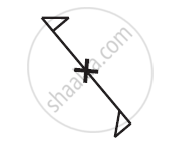Exercise 14.2 | Q 2.2 | Page 274

Give the order of rotational symmetry for given figure:Exercise 14.2 | Q 2.3 | Page 274

Give the order of rotational symmetry for given figure: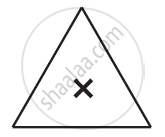Exercise 14.2 | Q 2.4 | Page 274

Give the order of rotational symmetry for each figure: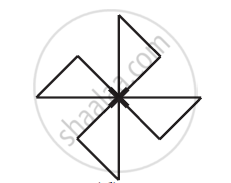Exercise 14.2 | Q 2.5 | Page 274

Give the order of rotational symmetry for given figure: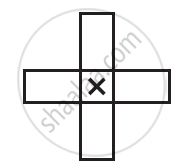Exercise 14.2 | Q 2.6 | Page 274

Give the order of rotational symmetry for given figure: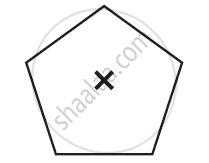Exercise 14.2 | Q 2.7 | Page 274

Give the order of rotational symmetry for given figure: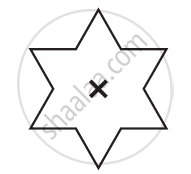Exercise 14.2 | Q 2.8 | Page 274

Give the order of rotational symmetry for given figure: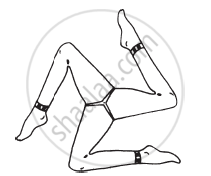Exercise 14.3 [Page 275]

### NCERT solutions for Class 7 Maths Chapter 14 Symmetry Exercise 14.3 [Page 275]

Exercise 14.3 | Q 1 | Page 275

Name any two figures that have both line symmetry and rotational symmetry.

Exercise 14.3 | Q 2.1 | Page 275

Draw, wherever possible, a rough sketch of a triangle with both line and rotational symmetries of order more than 1.

Exercise 14.3 | Q 2.2 | Page 275

Draw, wherever possible, a rough sketch of a triangle with only line symmetry and no rotational symmetry of order more than 1.

Exercise 14.3 | Q 2.2 | Page 275

Draw, wherever possible, a rough sketch of a quadrilateral with a rotational symmetry of order more than 1 but not a line symmetry.

Exercise 14.3 | Q 2.4 | Page 275

Draw, wherever possible, a rough sketch of a quadrilateral with line symmetry but not a rotational symmetry of order more than 1

Exercise 14.3 | Q 3 | Page 275

If a figure has two or more lines of symmetry, should it have rotational symmetry of order more than 1?

Exercise 14.3 | Q 4 | Page 275

Fill in the blanks:

 Shape Centre of Rotation Order of Rotation Angle of Rotation Square - - - Rectangle - - - Rhombus - - - Equilateral Triangle - - - Regular Hexagon - - - Circle - - - Semi-circle - - -

## Chapter 14: Symmetry

Exercise 14.1Exercise 14.2Exercise 14.3## NCERT solutions for Class 7 Maths chapter 14 - Symmetry

NCERT solutions for Class 7 Maths chapter 14 (Symmetry) include all questions with solution and detail explanation. This will clear students doubts about any question and improve application skills while preparing for board exams. The detailed, step-by-step solutions will help you understand the concepts better and clear your confusions, if any. Shaalaa.com has the CBSE Class 7 Maths solutions in a manner that help students grasp basic concepts better and faster.

Further, we at Shaalaa.com provide such solutions so that students can prepare for written exams. NCERT textbook solutions can be a core help for self-study and acts as a perfect self-help guidance for students.

Concepts covered in Class 7 Maths chapter 14 Symmetry are Concept of Symmetry, Lines of Symmetry for Regular Polygons, Concept of Rotational Symmetry, Line Symmetry and Rotational Symmetry, Concept of Reflection Symmetry.

Using NCERT Class 7 solutions Symmetry exercise by students are an easy way to prepare for the exams, as they involve solutions arranged chapter-wise also page wise. The questions involved in NCERT Solutions are important questions that can be asked in the final exam. Maximum students of CBSE Class 7 prefer NCERT Textbook Solutions to score more in exam.

Get the free view of chapter 14 Symmetry Class 7 extra questions for Class 7 Maths and can use Shaalaa.com to keep it handy for your exam preparation